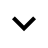Gordon Monro — Prints

# Gordon Monro — Alogos: Square and Alogos: Hexagonal

Computer-generated digital printsDescription

The ancient Greeks discovered that the ratio of the length of the diagonal of a square to the length of one of its sides is not equal to any ratio of whole numbers. The discovery is a triumph of pure thought, as no measurement can demonstrate this. The Greek mathematicians called this ratio and others like it "alogos", irrational.

The two patterns in Alogos: Square were made by superimposing two square grids, one rotated at 45 degrees. It is a consequence of the irrationality of the ratio between the side and the diagonal of a square that the two patterns in the diptych never repeat, however far they are extended.

The two patterns in Alogos: Hexagonal were made by superimposing two hexagonal grids, one rotated at 30 degrees. It is a consequence of the irrationality of the ratio between the length of the side of a cube and the length of one of its main diagonals that the two patterns in the diptych Alogos: Hexagonal never repeat, however far they are extended.

Alogos: Square and Alogos: Hexagonal are related to the print Alogos: Maze of Triangles, and Alogos: Hexagonal is also related to the Hexa series of prints.

Digital prints, each panel 70 x 70 cm. Editions of 5, 2017 and 2018.